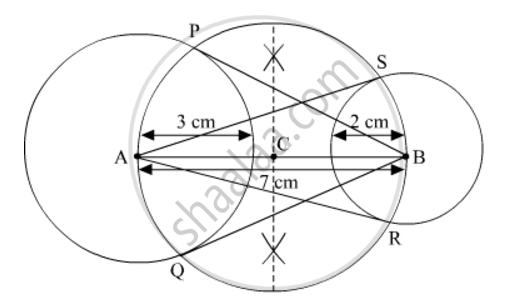# Draw a line segment AB of length 7 cm. Taking A as centre, draw a circle of radius 3 cm and taking B as centre, draw another circle of radius 2 cm. Construct tangents to each circle from the centre of the other circle.Steps - Mathematics

Draw a line segment AB of length 7 cm. Taking A as centre, draw a circle of radius 3 cm and taking B as centre, draw another circle of radius 2 cm. Construct tangents to each circle from the centre of the other circle.Steps

#### Solution

Steps of Construction

Step 1: Draw a line segment AB = 7 cm.

Step 2: With centre A and radius 3 cm, draw a circle.

Step 3: With centre B and radius 2 cm, draw a circle.

Step 4: Bisect the line AB. Let the mid-point of AB be C.

Step 5: Taking C as centre, draw a circle with AC as radius that intersect the circles at points P, Q, R and S.

Step 6: Join BP, BQ, AS and AR.Here, BP, BQ, AS and AR are the tangents to each circle from the centre of the other circle.

Concept: Construction of Tangents to a Circle
Is there an error in this question or solution?